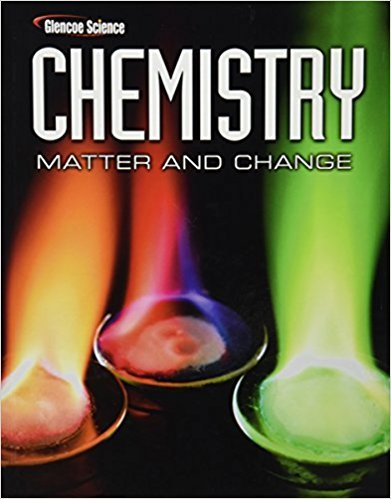×
×

# Synthesis of Phosgene Phosgene (COC l 2 ) is a toxic gasISBN: 9780078746376 131

## Solution for problem 90 Chapter 17

Chemistry: Matter & Change | 1st Edition

• Textbook Solutions
• 2901 Step-by-step solutions solved by professors and subject experts
• Get 24/7 help from StudySoup virtual teaching assistantsChemistry: Matter & Change | 1st Edition

4 5 0 331 Reviews
16
3
Problem 90

Synthesis of Phosgene Phosgene (COC l 2 ) is a toxic gas that is used in the manufacture of certain dyes, pharmaceuticals, and pesticides. Phosgene can be produced by the reaction between carbon monoxide and chlorine described by the equation CO(g) + C l 2 (g) COC l 2 (g). Initially 1.0000 mol CO and 1.0000 mol C l 2 are introduced into a 10.00-L reaction vessel. When equilibrium is established, both of their molar concentrations are found to be 0.0086 mol/L. What is the molar concentration of phosgene at equilibrium? What is K eq for the system?

Step-by-Step Solution:
Step 1 of 3
Step 2 of 3

Step 3 of 3

##### ISBN: 9780078746376

The full step-by-step solution to problem: 90 from chapter: 17 was answered by , our top Chemistry solution expert on 11/10/17, 05:56PM. The answer to “Synthesis of Phosgene Phosgene (COC l 2 ) is a toxic gas that is used in the manufacture of certain dyes, pharmaceuticals, and pesticides. Phosgene can be produced by the reaction between carbon monoxide and chlorine described by the equation CO(g) + C l 2 (g) COC l 2 (g). Initially 1.0000 mol CO and 1.0000 mol C l 2 are introduced into a 10.00-L reaction vessel. When equilibrium is established, both of their molar concentrations are found to be 0.0086 mol/L. What is the molar concentration of phosgene at equilibrium? What is K eq for the system?” is broken down into a number of easy to follow steps, and 98 words. This textbook survival guide was created for the textbook: Chemistry: Matter & Change, edition: 1. Chemistry: Matter & Change was written by and is associated to the ISBN: 9780078746376. This full solution covers the following key subjects: . This expansive textbook survival guide covers 24 chapters, and 2831 solutions. Since the solution to 90 from 17 chapter was answered, more than 230 students have viewed the full step-by-step answer.

Unlock Textbook Solution